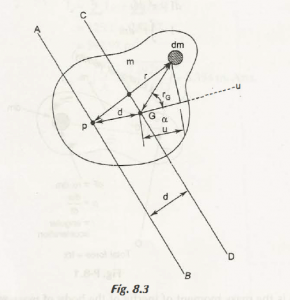Considering the Fig. 8.3,Suppose AB and CD are two parallel lines. AB passes through any point P of a rigid body and CD passes through its centre of mass, G.

From trigonometry, we get the calculation as-

r2G + d2 +r2/ 2rG d

= r2G + d2 +r2/ 2rG d

2r2G+ d = r2G + d2 – r2

r2 = r2G + d2 + 2rGd

Now,

I = 2dm

= 2G + d2 + 2rGd } dm

=2Gdm + d22Gdm

= IG + d2m + 2d dm

= IG + md2 + 0                                                (Since, dm = 0)

Hence,

I = IG + md2

Similarly, we can calculate,

K2 = K2G + d2

Where,

KG is the Radius of Gyration

Links of Next Mechanical Engineering Topics:-### Customer Reviews

My Homework Help
Rated 5.0 out of 5 based on 510 customer reviews at